01signal.com

# Conversion between Galois and Fibonacci polynomials of Linear-Feedback Shift Register

### Introduction

This page can be summarized in one sentence: If you want to convert an LFSR's polynomial from Galois form to Fibonacci form, reverse the order of the coefficients.

For example, this is the drawing for the LFSR that is often used in scramblers: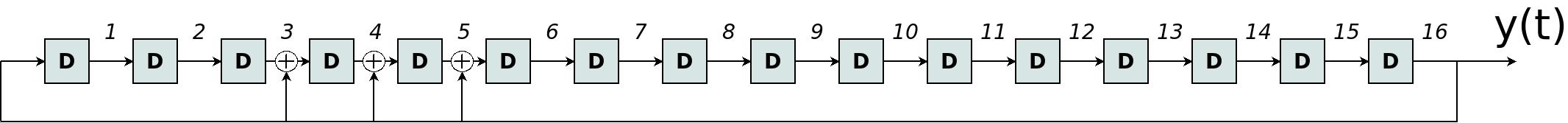Each block that is marked with D is a delay of one clock cycle. The representation of this LFSR as a Galois polynomial is:

G(x) = x16 + x5 + x4 + x3 + 1

Note that the exponents in the polynomial (3, 4, and 5) reflect the number of delay elements on the left side of each XOR.

The same LFSR can be implemented in Fibonacci form, as shown in this drawing: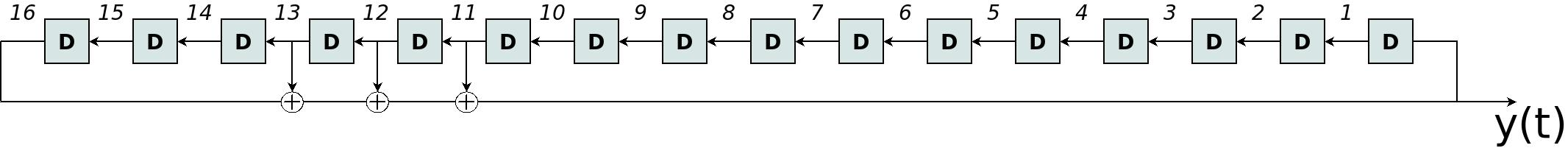Despite the similarity between these two drawings, the directions of the arrows are reversed. The implementations of the LFSRs are completely different.

The Fibonacci polynomial (or feedback polynomial) is this:

F(x) = x16 + x13 + x12 + x11 + 1

The degree of the polynomial is n=16, so each xi in G(x) is represented in F(x) by xn-i. The rest of this page shows why this is not a coincidence.

### LFSR and digital filters

The theory behind digital filters (e.g. FIRs and IIRs) is usually applied on the field of complex numbers. However, it's also possible to apply this theory on GF(2), which is the finite field (Galois Field) that consists of two numbers: 0 and 1. There are a few things to note:

• The plus operator in this field is equivalent to a XOR.
• All multiplications involve only integers. In fact, multiplying with an even number is equivalent to multiplying with 0. Likewise, multiplying with an odd number is equivalent to multiplying with 1. It doesn't matter if this number is positive or negative.
• Because of this rule with multiplications, plus and minus mean the same. Both can be replaced with ⊕ (which means XOR).

The basic theory of digital filters only requires that the system is Linear time-invariant (LTI). An LFSR meets these requirements, because XOR is linear, and the LFSR's behavior is time-invariant.

The most interesting consequence is that the z-transform can be used to analyze LFSRs. For example, consider a this drawing: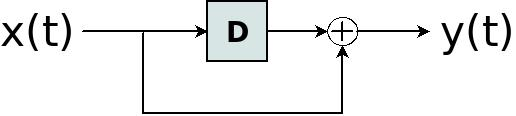This can be described with the following equation:

y(t) = x(t) ⊕ x(t-1)

t is an integer that represents time.

It's possible to treat this as a FIR, and write the z-transform of this equation:

Y(z) = X(z) + X(z)z-1=X(z) (1 + z-1)

Accordingly, the "impulse response" can be defined as usual: H(z) = Y(z)/X(z), or simply:

H(z) = 1 + z-1

But what if we put two of these filters one after the other? What is the "impulse response" of this filter?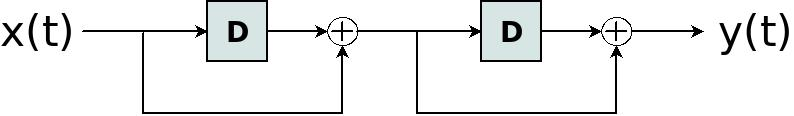The theory for digital filters has a simple answer:

H2(z) = H(z)H(z) = (1 + z-1)(1 + z-1) = 1 + 2z-1 + z-2

Recall that with GF(2), a multiplication with an even number is like a multiplication with zero. Accordingly,

H2(z) = 1 + 2z-1 + z-2 = 1 + 0z-1 + z-2=1 + z-2

which corresponds to this: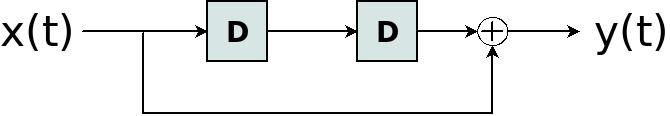It's not easy to see that the filters that are shown in the two last drawings are equivalent. But both filters produce the same y(t) when they are fed with the same x(t). So the filters are the same, even though the values that are stored in the second delay element are not the same.

This example shows the advantage of using the z-transform (and similar techniques with polynomials) to analyze logic filters.

I should mention that the symbols D or D-1 are often used instead of z-1. In fact, the use of x in Galois and Fibonacci polynomials has the same meaning as z-1. All these symbols mean a delay.

### The Galois LFSR

In order to find the Fibonacci representation, I'll start with looking at the Galois LFSR.

Consider the drawing for the Galois LFSR from above. The row of delay lines and taps can be viewed as a FIR filter, which I shall denote A(z). The Galois LFSR is hence equivalent to this: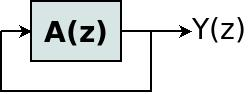Due to the feedback, the equation for Y(z) is:

Y(z) = Y(z)A(z)

So what is the connection between this FIR's parameters and Galois polynomial? Let's write the general form of the Galois polynomial:

G(x) = xn + gn-1xn-1+ gn-2xn-2+…+ g0

Note that gn doesn't appear in this expression, in order to simplify the mathematics below. Besides, gn always equals 1.

The relation between G(x) and A(z) is as follows:

A(z) = gn-1z-1+gn-2z-2+…+g1z1-n+g0z-n

Why is this correct? Let's take the Galois LFSR from the drawing above, and show how this expression works.

First, let's look at the XOR that corresponds to g5. The number 5 means that there are five delay elements on the left side of this XOR. But the exponent of z depends on the number of delay elements between the XOR and the FIR's output. These are the delay elements on the right side of the XOR. There are 16 delay elements in total. Therefore, g5 appears in A(z) as g5z-11. This matches the gn-iz-i pattern.

Another way to explain this is to look at A(z) as a FIR in the time domain. This FIR's input is x(t) and its output is y(t):

y(t) = x(t–16) ⊕ x(t–13) ⊕ x(t–12) ⊕ x(t–11)

Once again, t is an integer that represents time. This expression represents the Galois LFSR without the feedback. This FIR's transfer function is:

A(z) = Y(z)/X(z) = z-16 + z-13 + z-12 + z-11

This is consistent with the general expression for A(z) with G(x) = x16 + x5 + x4 + x3 + 1.

### The Fibonacci equivalent

An LFSR in Fibonacci form is represented by the following polynomial:

F(x) = fnxn+fn-1xn-1+…+f1x + 1

Note that the f0 doesn't appear in this polynomial because it always equals 1. This is similar to gn not appearing in G(x).

The implementation of this polynomial is:

y(t) = f1y(t-1) + f2y(t-2) +…+ fny(t-n)

The z transform is accordingly:

Y(z) = f1Y(z)z-1+f2Y(z)z-2+…+fnY(z)z-n

Let's define B(z) as follows:

B(z) = f1z-1+f2z-2+…+fnz-n

Y(z) can consequently be written as:

Y(z) = Y(z)B(z)

Recall from above that Y(z) = Y(z)A(z). Hence if the Fibonacci form of the LFSR behaves the same as the LFSR that is given by the Galois polynomial, it follows that A(z) = B(z). Which also means that:

fi=gn-i (i=1,2, … , n)

So as I said from the start: In order to convert an LFSR's polynomial from Galois form to Fibonacci form, reverse the order of the coefficients.

### The LFSR's inverse filter

Once again, this is the Fibonacci implementation of the LFSR:

y(t) = f1y(t-1) + f2y(t-2) +…+ fny(t-n)

but this expression can also be rewritten as follows:

y(t) + f1y(t-1) + f2y(t-2) +…+ fny(t-n) = 0

(recall that + and – are the same in GF(2))

So the Fibonacci polynomial's coefficients also tell us how to perform XOR on the values of y(t-i) in order to always get the value zero. In other words, this describes an interesting FIR.

The z transform of the last expression is:

Y(z) + f1Y(z)z-1+f2Y(z)z-2+…+fnY(z)z-n = 0

Or, equivalently:

Y(z)(1+ f1z-1+f2z-2+…+fnz-n) = Y(z)H(z) = 0

Y(z)H(z) is the output when the filter H(z) is applied to Y(z). And this output is zero.

Hence H(z) is the transfer function of a FIR filter. If you feed this FIR with the output of the LFSR that is defined by F(x), the output will be zero all the time. From the definition of B(z) above, this FIR's transfer function is:

H(z) = 1 + f1z-1+f2z-2+…+fnz-n

Or in the time domain, if the input is x(t) and the output is y(t):

y(t) = x(t) ⊕ f1x(t-1) ⊕ f2x(t-2) ⊕ … ⊕ fnx(t-n)

If x(t) is the output of the LFSR that is defined by F(x), y(t) is zero for all t.